## Writeup-北邮新生赛MRCTF-Web题：套娃

2020/10/16 18:29:41 文章标签: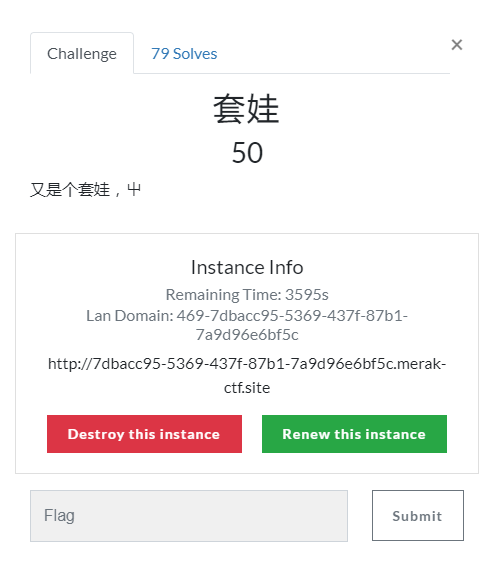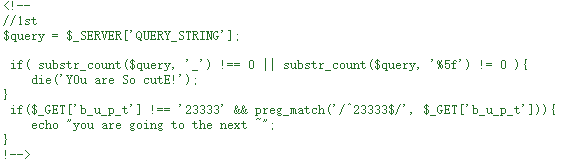//1st
$query =$_SERVER['QUERY_STRING'];

if( substr_count($query, '_') !== 0 || substr_count($query, '%5f') != 0 ){
die('Y0u are So cutE!');
}
if($_GET['b_u_p_t'] !== '23333' && preg_match('/^23333$/', $_GET['b_u_p_t'])){ echo "you are going to the next ~"; }  首先将$_SERVER['QUERY_STRING']的值赋给变量$query 关于$_SERVER['QUERY_STRING']获取的值：

1，http://localhost/aaa/ (打开aaa中的index.php)

$_SERVER[‘QUERY_STRING’] = “”;$_SERVER[‘REQUEST_URI’] = “/aaa/”;
$_SERVER[‘SCRIPT_NAME’] = “/aaa/index.php”;$_SERVER[‘PHP_SELF’] = “/aaa/index.php”;

2，http://localhost/aaa/?p=222 (附带查询)

$_SERVER[‘QUERY_STRING’] = “p=222”;$_SERVER[‘REQUEST_URI’] = “/aaa/?p=222”;
$_SERVER[‘SCRIPT_NAME’] = “/aaa/index.php”;$_SERVER[‘PHP_SELF’] = “/aaa/index.php”;

3，http://localhost/aaa/index.php?p=222&q=333

$_SERVER[‘QUERY_STRING’] = “p=222&q=333”;$_SERVER[‘REQUEST_URI’] = “/aaa/index.php?p=222&q=333”;
$_SERVER[‘SCRIPT_NAME’] = “/aaa/index.php”;$_SERVER[‘PHP_SELF’] = “/aaa/index.php”;

$_SERVER[“QUERY_STRING”] 获取查询 语句，实例中可知，获取的是?后面的值$_SERVER[“REQUEST_URI”] 获取 http://localhost 后面的值，包括/
$_SERVER[“SCRIPT_NAME”] 获取当前脚本的路径，如：index.php$_SERVER[“PHP_SELF”] 当前正在执行脚本的文件名

if( substr_count($query, '_') !== 0 || substr_count($query, '%5f') != 0 )


if($_GET['b_u_p_t'] !== '23333' && preg_match('/^23333$/', $_GET['b_u_p_t']))  我们需要同时满足两个条件，其中preg_match函数用于匹配正则表达式，这里需要通过get提交参数b_u_p_t，但是参照第一层提到的substr_count函数，所以get参数不能带下划线，这时我们可以用点来代替下划线，同时用%0a来进行过滤正则表达式的条件，最终get传递的参数为?b.u.p.t=23333%0a根据提示进入secrettw.php,仍然是标准套路，提示需要本地才能访问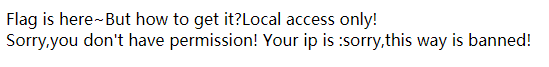右键查看源码，发现一大堆注释，乍一看像乱码，实际上ctf里的注释大家也都知道是怎么回事，肯定不是毫无意义的，百度一查，发现是一种叫jother的编码，直接复制粘贴到浏览器控制台即可解码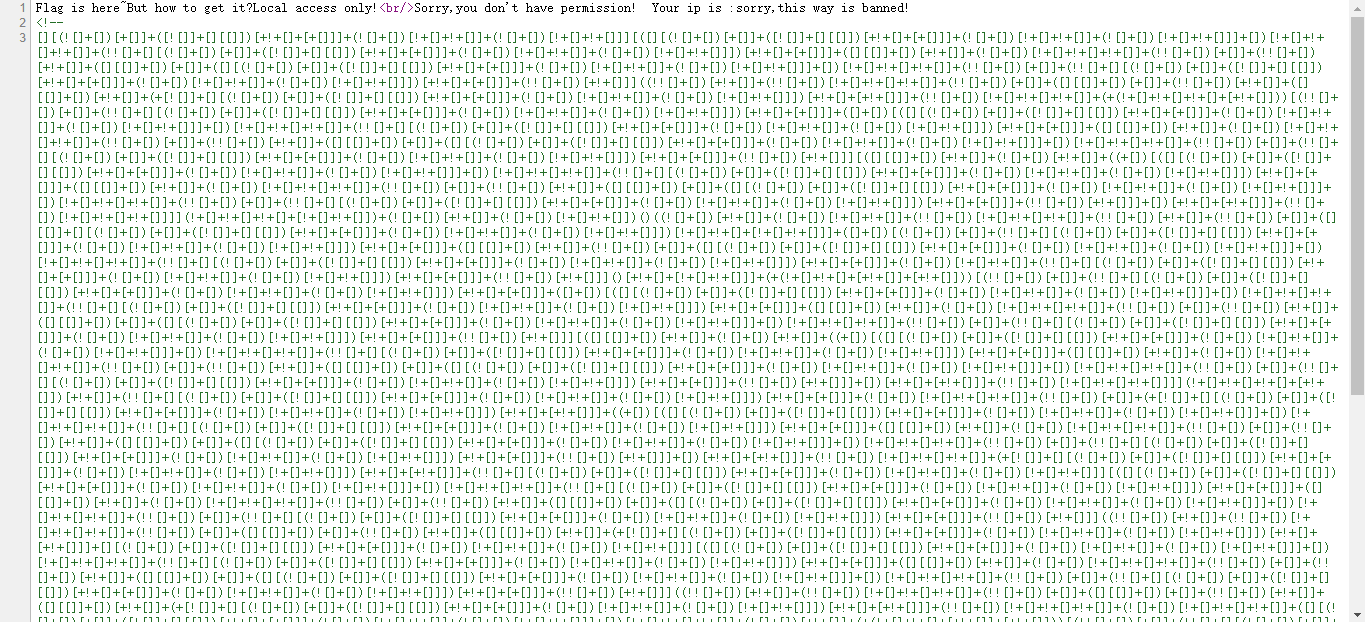执行后弹出了一段alert信息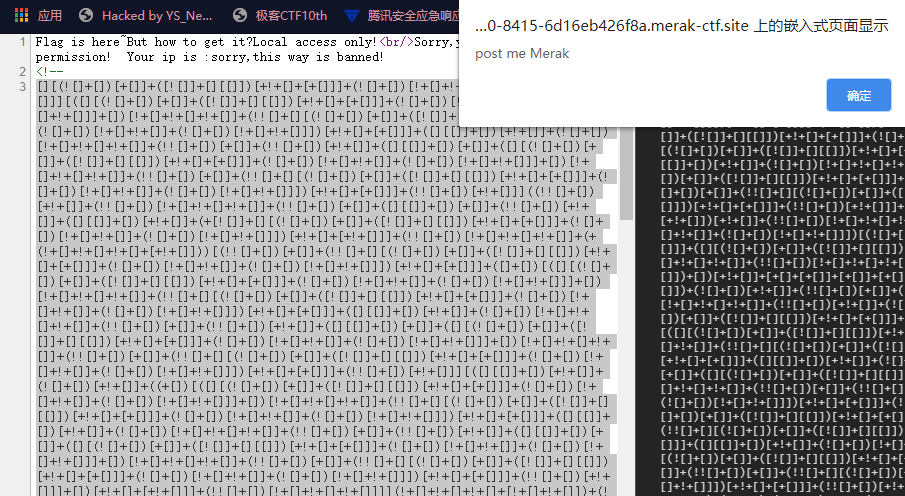根据提示用hackbar通过POST提交一个Merak参数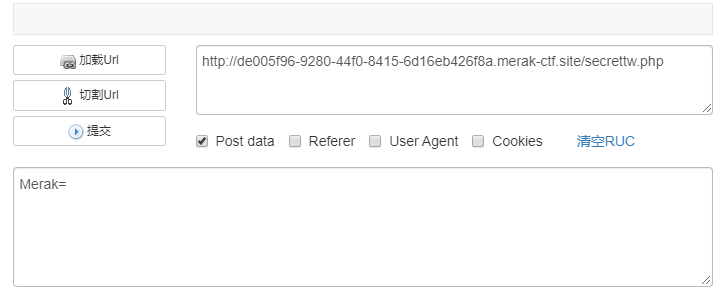返回了一段代码，应该是secrettw.php的部分源码高亮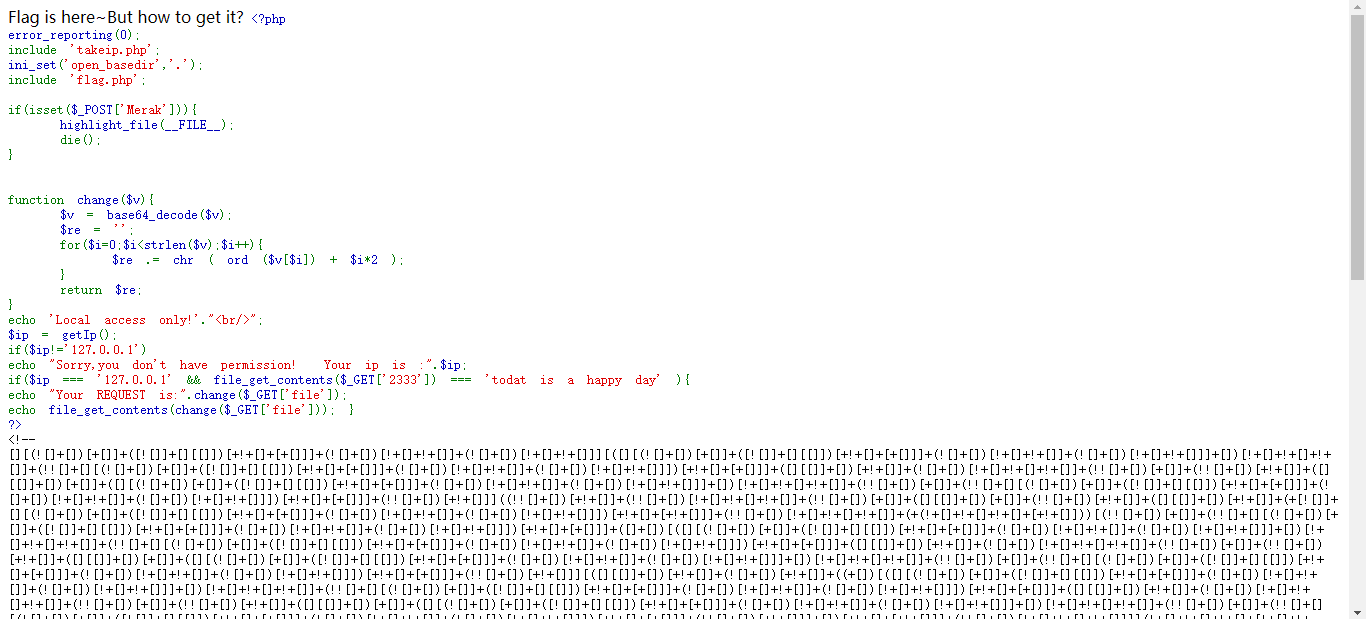源码如下 Flag is here~But how to get it? <?php error_reporting(0); include 'takeip.php'; ini_set('open_basedir','.'); include 'flag.php'; if(isset($_POST['Merak'])){
highlight_file(__FILE__);
die();
}

function change($v){$v = base64_decode($v);$re = '';
for($i=0;$i<strlen($v);$i++){
$re .= chr ( ord ($v[$i]) +$i*2 );
}
return $re; } echo 'Local access only!'."<br/>";$ip = getIp();
if($ip!='127.0.0.1') echo "Sorry,you don't have permission! Your ip is :".$ip;
if($ip === '127.0.0.1' && file_get_contents($_GET['2333']) === 'todat is a happy day' ){
echo "Your REQUEST is:".change($_GET['file']); echo file_get_contents(change($_GET['file'])); }
?>


if($ip === '127.0.0.1' && file_get_contents($_GET['2333']) === 'todat is a happy day' )


echo "Your REQUEST is:".change($_GET['file']); echo file_get_contents(change($_GET['file']));


$v = base64_decode($v);
$re = ''; for($i=0;$i<strlen($v);$i++){$re .= chr ( ord ($v[$i]) + $i*2 ); } return$re;
}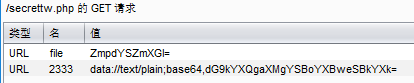burpsuite改包放行后返回页面，右键查看源码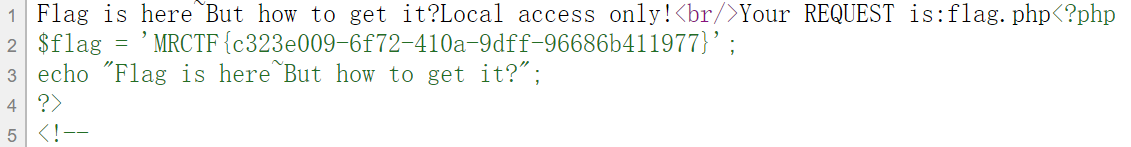暂无相关的数据...